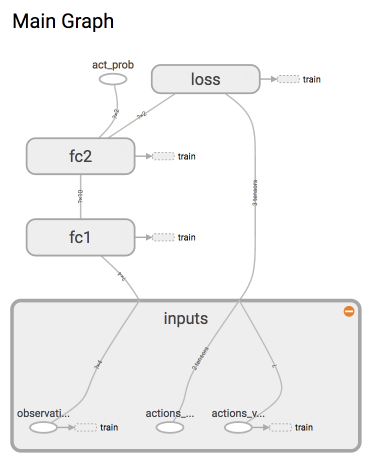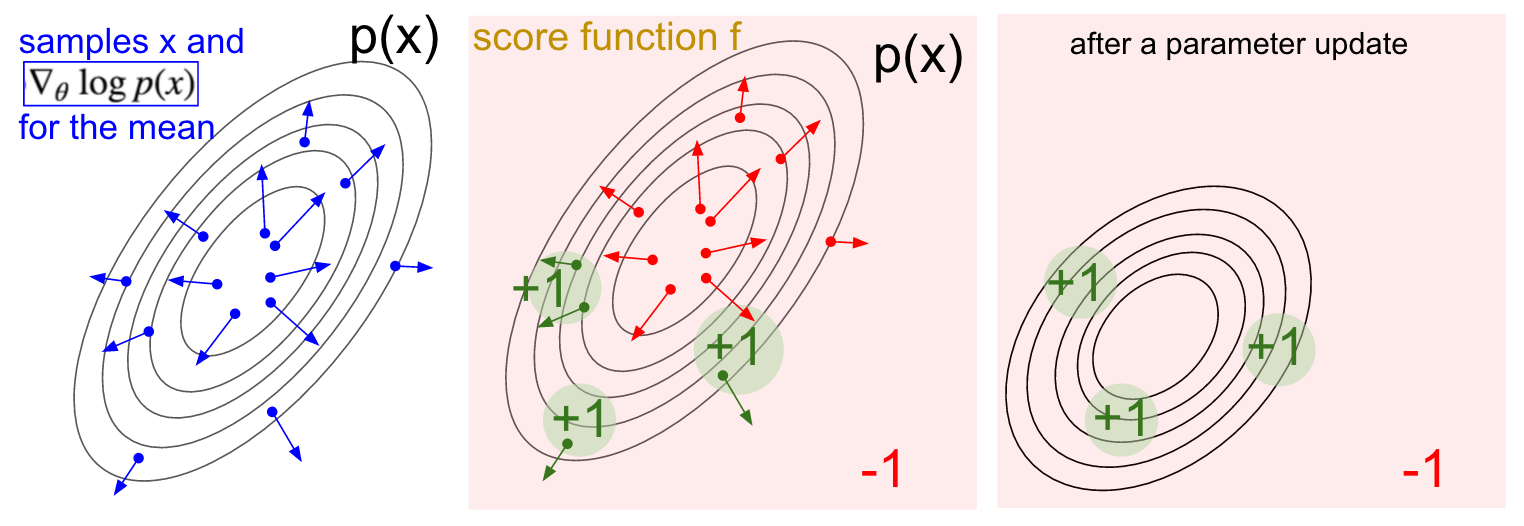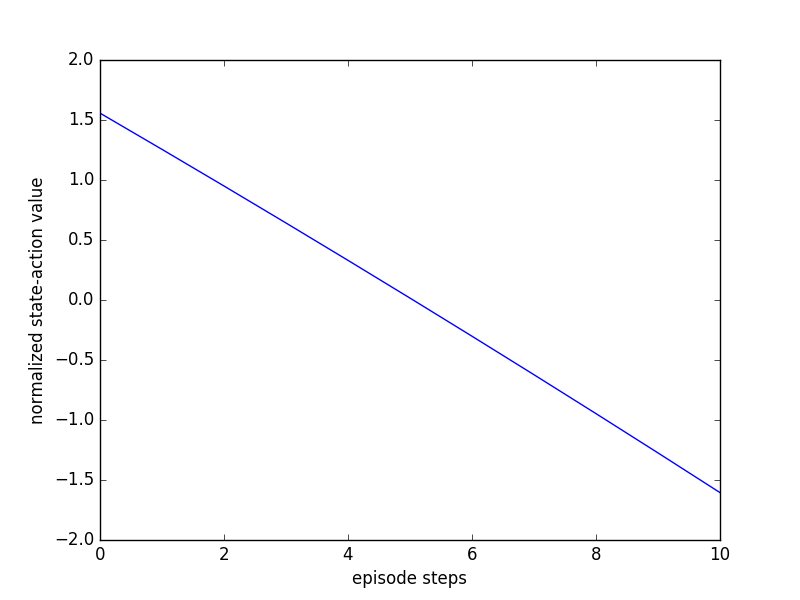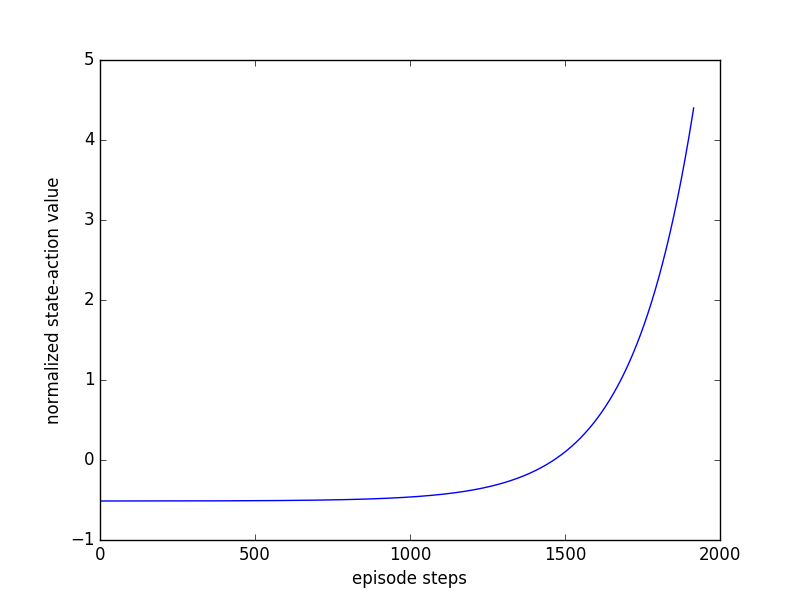## 要代码主结构 ¶

class PolicyGradient:
# 初始化 (有改变)
def __init__(self, n_actions, n_features, learning_rate=0.01, reward_decay=0.95, output_graph=False):

# 建立 policy gradient 神经网络 (有改变)
def _build_net(self):

# 选行为 (有改变)
def choose_action(self, observation):

# 存储回合 transition (有改变)
def store_transition(self, s, a, r):

# 学习更新参数 (有改变)
def learn(self, s, a, r, s_):

# 衰减回合的 reward (新内容)
def _discount_and_norm_rewards(self):


## 初始化 ¶

class PolicyGradient:
def __init__(self, n_actions, n_features, learning_rate=0.01, reward_decay=0.95, output_graph=False):
self.n_actions = n_actions
self.n_features = n_features
self.lr = learning_rate     # 学习率
self.gamma = reward_decay   # reward 递减率

self.ep_obs, self.ep_as, self.ep_rs = [], [], []    # 这是我们存储 回合信息的 list

self._build_net()   # 建立 policy 神经网络

self.sess = tf.Session()

if output_graph:    # 是否输出 tensorboard 文件
# \$ tensorboard --logdir=logs
# http://0.0.0.0:6006/
# tf.train.SummaryWriter soon be deprecated, use following
tf.summary.FileWriter("logs/", self.sess.graph)

self.sess.run(tf.global_variables_initializer())



## 建立 Policy 神经网络 ¶class PolicyGradient:
def __init__(self, n_actions, n_features, learning_rate=0.01, reward_decay=0.95, output_graph=False):
...
def _build_net(self):
with tf.name_scope('inputs'):
self.tf_obs = tf.placeholder(tf.float32, [None, self.n_features], name="observations")  # 接收 observation
self.tf_acts = tf.placeholder(tf.int32, [None, ], name="actions_num")   # 接收我们在这个回合中选过的 actions
self.tf_vt = tf.placeholder(tf.float32, [None, ], name="actions_value") # 接收每个 state-action 所对应的 value (通过 reward 计算)

# fc1
layer = tf.layers.dense(
inputs=self.tf_obs,
units=10,   # 输出个数
activation=tf.nn.tanh,  # 激励函数
kernel_initializer=tf.random_normal_initializer(mean=0, stddev=0.3),
bias_initializer=tf.constant_initializer(0.1),
name='fc1'
)
# fc2
all_act = tf.layers.dense(
inputs=layer,
units=self.n_actions,   # 输出个数
activation=None,    # 之后再加 Softmax
kernel_initializer=tf.random_normal_initializer(mean=0, stddev=0.3),
bias_initializer=tf.constant_initializer(0.1),
name='fc2'
)

self.all_act_prob = tf.nn.softmax(all_act, name='act_prob')  # 激励函数 softmax 出概率

with tf.name_scope('loss'):
# 最大化 总体 reward (log_p * R) 就是在最小化 -(log_p * R), 而 tf 的功能里只有最小化 loss
neg_log_prob = tf.nn.sparse_softmax_cross_entropy_with_logits(logits=all_act, labels=self.tf_acts) # 所选 action 的概率 -log 值
# 下面的方式是一样的:
# neg_log_prob = tf.reduce_sum(-tf.log(self.all_act_prob)*tf.one_hot(self.tf_acts, self.n_actions), axis=1)
loss = tf.reduce_mean(neg_log_prob * self.tf_vt)  # (vt = 本reward + 衰减的未来reward) 引导参数的梯度下降

with tf.name_scope('train'):## 选行为 ¶

class PolicyGradient:
def __init__(self, n_actions, n_features, learning_rate=0.01, reward_decay=0.95, output_graph=False):
...
def _build_net(self):
...
def choose_action(self, observation):
prob_weights = self.sess.run(self.all_act_prob, feed_dict={self.tf_obs: observation[np.newaxis, :]})    # 所有 action 的概率
action = np.random.choice(range(prob_weights.shape), p=prob_weights.ravel())  # 根据概率来选 action
return action


## 存储回合 ¶

class PolicyGradient:
def __init__(self, n_actions, n_features, learning_rate=0.01, reward_decay=0.95, output_graph=False):
...
def _build_net(self):
...
def choose_action(self, observation):
...
def store_transition(self, s, a, r):
self.ep_obs.append(s)
self.ep_as.append(a)
self.ep_rs.append(r)


## 学习 ¶

class PolicyGradient:
def __init__(self, n_actions, n_features, learning_rate=0.01, reward_decay=0.95, output_graph=False):
...
def _build_net(self):
...
def choose_action(self, observation):
...
def store_transition(self, s, a, r):
...
def learn(self):
# 衰减, 并标准化这回合的 reward
discounted_ep_rs_norm = self._discount_and_norm_rewards()   # 功能再面

# train on episode
self.sess.run(self.train_op, feed_dict={
self.tf_obs: np.vstack(self.ep_obs),  # shape=[None, n_obs]
self.tf_acts: np.array(self.ep_as),  # shape=[None, ]
self.tf_vt: discounted_ep_rs_norm,  # shape=[None, ]
})

self.ep_obs, self.ep_as, self.ep_rs = [], [], []    # 清空回合 data
return discounted_ep_rs_norm    # 返回这一回合的 state-action value


vt = RL.learn() # 学习, 输出 vt, 我们下节课讲这个 vt 的作用

if i_episode == 0:
plt.plot(vt)    # plot 这个回合的 vt
plt.xlabel('episode steps')
plt.ylabel('normalized state-action value')
plt.show()“请重视我这回合开始时的一系列动作, 因为前面一段时间杆子还没有掉下来. 而且请惩罚我之后的一系列动作, 因为后面的动作让杆子掉下来了” 或者是

“我每次都想让这个动作在下一次增加被做的可能性 (grad(log(Policy))), 但是增加可能性的这种做法是好还是坏呢? 这就要由 vt 告诉我了, 所以后段时间的 增加可能性 做法并没有被提倡, 而前段时间的 增加可能性 做法是被提倡的.”class PolicyGradient:
def __init__(self, n_actions, n_features, learning_rate=0.01, reward_decay=0.95, output_graph=False):
...
def _build_net(self):
...
def choose_action(self, observation):
...
def store_transition(self, s, a, r):
...
def learn(self):
...
def _discount_and_norm_rewards(self):
# discount episode rewards
discounted_ep_rs = np.zeros_like(self.ep_rs)
for t in reversed(range(0, len(self.ep_rs))):